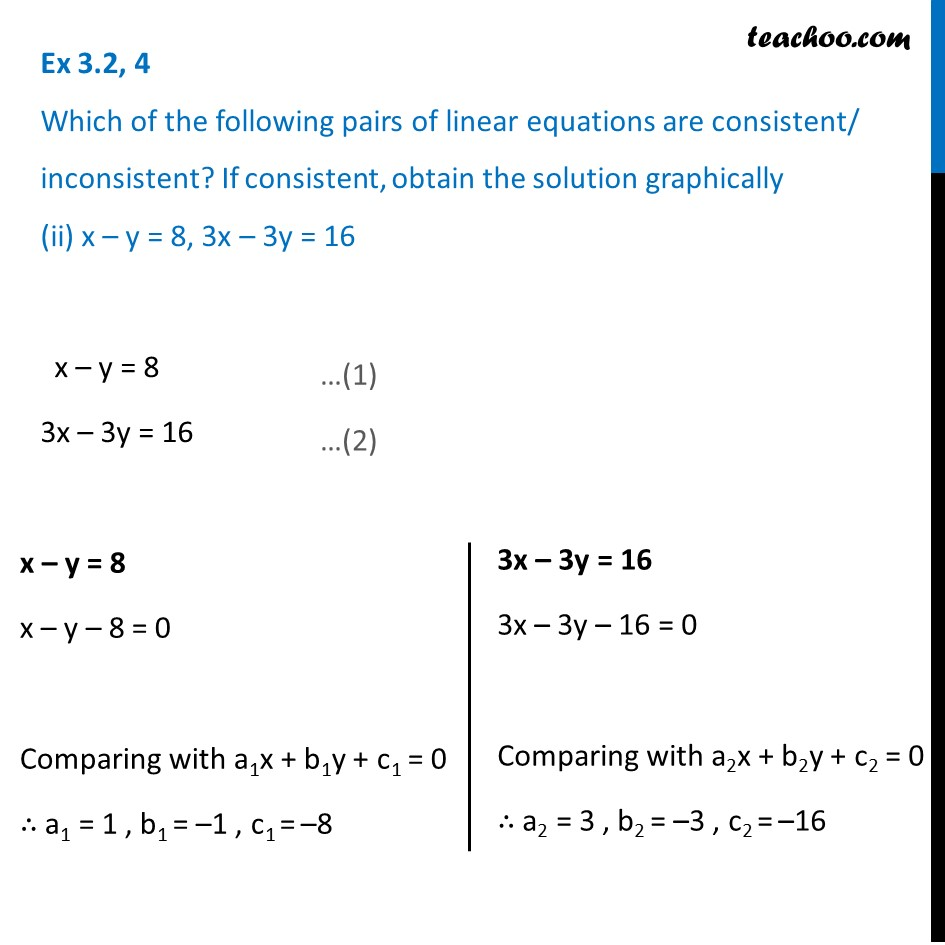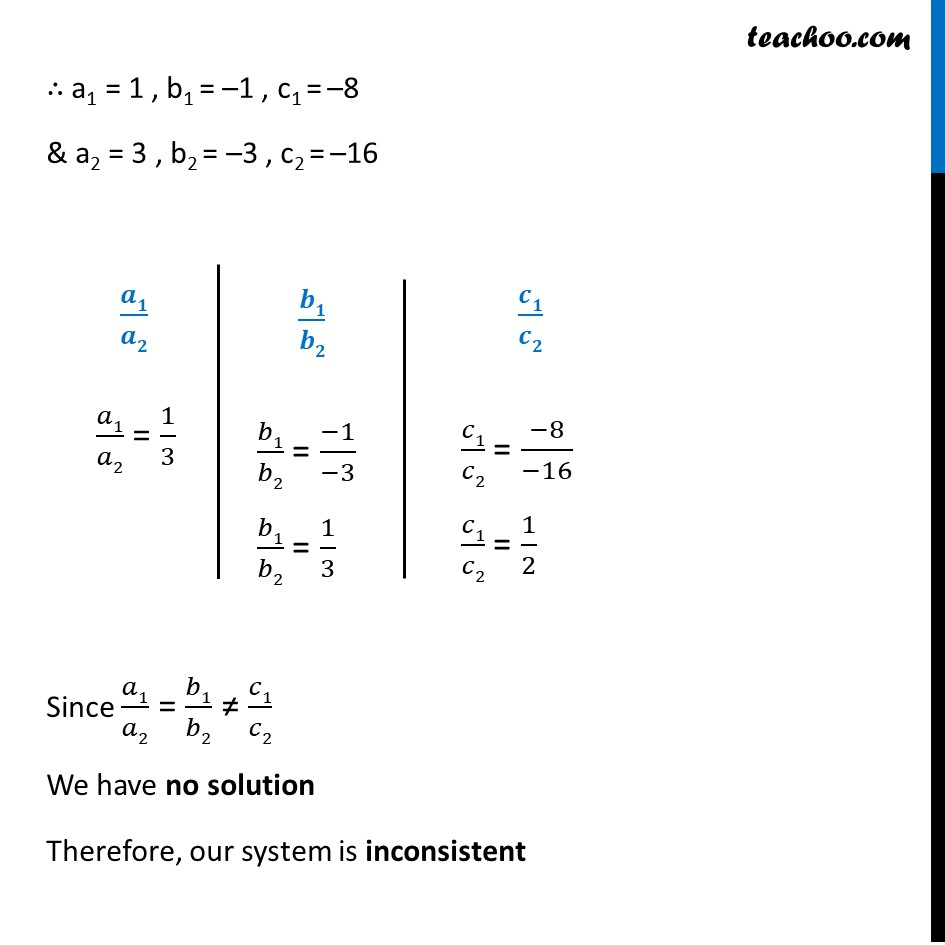1. Chapter 3 Class 10 Pair of Linear Equations in Two Variables (Term 1)
2. Serial order wise
3. Ex 3.2

Transcript

Ex 3.2, 4 Which of the following pairs of linear equations are consistent/ inconsistent? If consistent, obtain the solution graphically (ii) x – y = 8, 3x – 3y = 16 x – y = 8 3x – 3y = 16 x – y = 8 x – y – 8 = 0 Comparing with a1x + b1y + c1 = 0 ∴ a1 = 1 , b1 = –1 , c1 = –8 3x – 3y = 16 3x – 3y – 16 = 0 Comparing with a2x + b2y + c2 = 0 ∴ a2 = 3 , b2 = –3 , c2 = –16 ∴ a1 = 1 , b1 = –1 , c1 = –8 & a2 = 3 , b2 = –3 , c2 = –16 𝒂𝟏/𝒂𝟐 𝑎1/𝑎2 = 1/3 𝒃𝟏/𝒃𝟐 𝑏1/𝑏2 = (−1)/(−3) 𝑏1/𝑏2 = 1/3 𝒄𝟏/𝒄𝟐 𝑐1/𝑐2 = (−8)/(−16) 𝑐1/𝑐2 = 1/2 Since 𝑎1/𝑎2 = 𝑏1/𝑏2 ≠ 𝑐1/𝑐2 We have no solution Therefore, our system is inconsistent